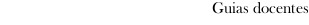Educational guide 2018_19Escola de Enxeñaría Industrial
 Degree in Mechanical EngineeringSubjectsMathematics: Algebra and statisticsContents
 Topic Sub-topic Preliminaries The field of complex numbers. Matrices, determinants and systems of linear equations. Definition and types of matrices. Matrices operations. Elementary transformations, row echelon forms, rank of a matrix. Inverse and determinant of a square matrix. Consistency of systems of linear equations and their solutions. Vector spaces and linear maps. Vector space. Subspaces. Linear independence, basis and dimension. Coordinates, change of basis. Basic notions on linear maps. Eigenvalues and eigenvectors. Definition of eigenvalue and eigenvector of a square matrix. Diagonalization of matrices by similarity transformation. Applications of eigenvalues and eigenvectors. Vector spaces with scalar product and quadratic forms. Vectorial spaces with scalar product. Associated norm and properties. Orthogonality. Gram-Schmidt orthonormalization process. Orthogonal diagonalization of a real and symmetric matrix. Quadratic forms. Probability. Concept and properties. Conditional probability and independence of events. Bayes Theorem. Discrete random variables and continuous random variables. Definition of random variable. Types of random variables. Distribution function. Discrete random variables. Continuous random variables. Characteristics of a random variable. Main distributions: Binomial, Geometric, Poisson, Hypergeometric, Uniform, Exponential, Normal. Central Limit Theorem. Statistical inference. General concepts. Sampling distributions. Point estimation. Confidence intervals. Tests of hypotheses. Regression. Scatterplot. Correlation. Linear regression: regression line. Inference about the parameters of the regression line.
 Universidade de Vigo            | Reitoría | Campus Universitario | C.P. 36.310 Vigo (Pontevedra) | Spain | Tlf: +34 986 812 000